# Gas Engineering Guide

### Gas Piping – Required Gas Piping Size

The size of the iron-piping outlet for any gas appliance shall not be less than one-half (1/2) inch, for standard gas piping pressure.

When an additional appliance is to be served through present gas piping, capacity of the existing piping shall be properly sized and replaced with larger piping if necessary. The size of gas piping depends on the following factors:

• Allowable loss in pressure from point of delivery to equipment
• Maximum gas demand
• Length of piping and number of fittings
• Specific gravity of the gas
• Diversity factor
• Forseeable future demand

To determine the size of any section of pipe in a system, proceed as follows:

A. Calculate the gas demand in cubic feet per hour of the appliance(s) to be attached to each outlet (see Required Gas Supply to calculate demand)
B. Measure the length of pipe from the meter to the most remote outlet
C. In Appendix B, select the distance, or the next longer distance if the table does not give the exact length
D. Use this horizontal line to locate all gas demand figures for this particular system of piping
E. Starting at the most remote outlet, find the horizontal line just selected, determine the gas demand for the outlet (if the exact figure of demand is not shown, choose the next larger figure in the horizontal line)
F. Above the demand figure will be found the correct size of gas piping
G. Proceed in a similar manner for each outlet and each section of gas piping

EXAMPLE OF LOW PRESSURE PIPE SIZING: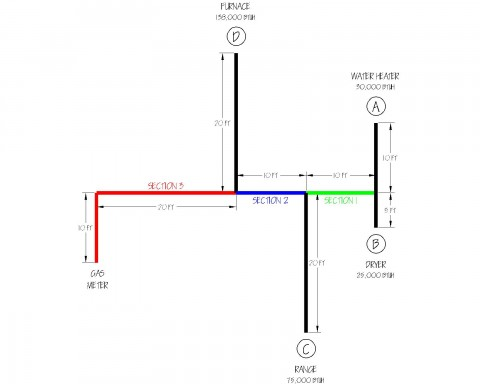SOLUTION:
Natural Gas (1050 Btu/CF)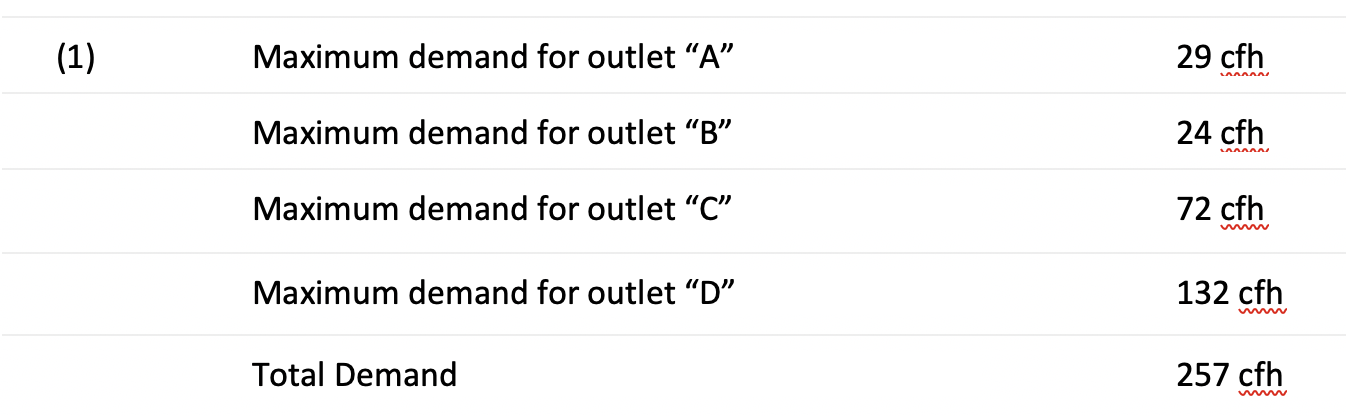(2) The length of pipe from the gas meter to the remote outlet (Outlet “A”) is 60 ft. THIS IS THE ONLY DISTANCE USED FOR SIZING PIPE.

(3) Using a low pressure sizing table in the Uniform Plumbing Code or other gas reference table: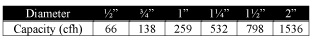(4) Pipe Sizing
• Outlet “A” supplying 29 cfh requires 1/2” pipe
• Outlet “B” supplying 24 cfh requires 1/2” pipe
• Section 1 supplying outlets “A” and “B”, 53 cfh, requires 1/2” pipe
• Outlet “C” supplying 72 cfh requires 3/4” pipe
• Section 2 supplying outlets “A”, “B”, and “C”, 125 cfh, requires 3/4” pipe
• Outlet “D” supplying 130 cfh requires 3/4” pipe
• Section 3 supplying outlets “A”, “B”, “C”, and “D”, 255 cfh, requires 1” pipe

Propane Gas (2520 Btu/CF)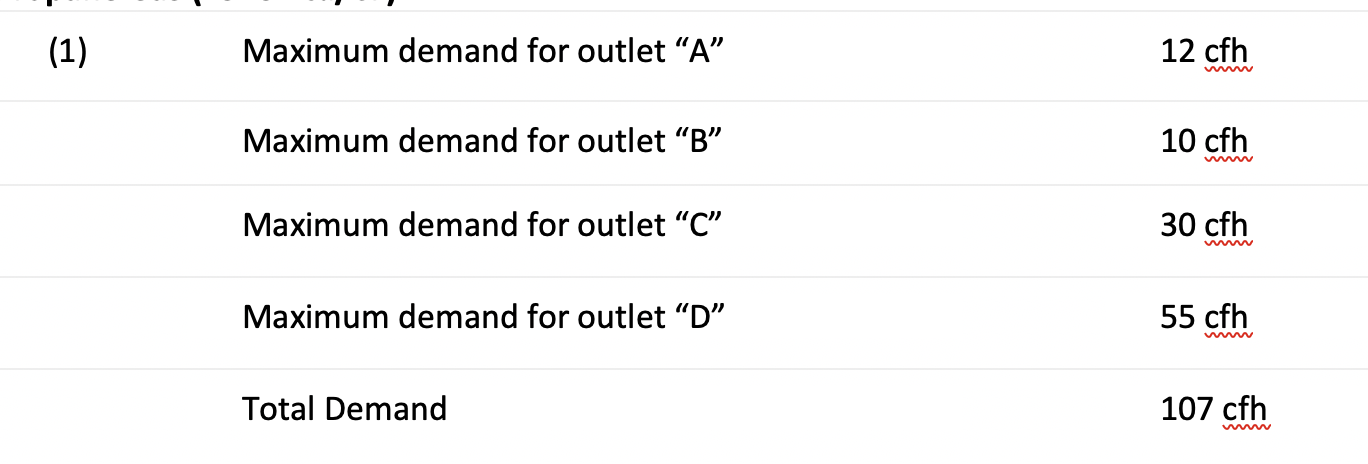(2) The length of pipe from the gas meter to the remote outlet (Outlet “A”) is 60 ft. THIS IS THE ONLY DISTANCE USED FOR SIZING PIPE.(3) Using a low pressure sizing table in the Uniform Plumbing Code or other gas reference table:

(3) Using a low pressure sizing table in the Uniform Plumbing Code or other gas reference table: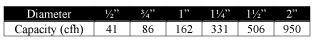(4) Pipe Sizing
• Outlet “A” supplying 12 cfh requires 1/2” pipe
• Outlet “B” supplying 10 cfh requires 1/2” pipe
• Section 1 supplying outlets “A” and “B”, 22 cfh, requires 1/2” pipe
• Outlet “C” supplying 30 cfh requires 1/2” pipe
• Section 2 supplying outlets “A”, “B”, and “C”, 52 cfh, requires 3/4” pipe
• Outlet “D” supplying 55 cfh requires 3/4” pipe
• Section 3 supplying outlets “A”, “B”, “C”, and “D”, 107 cfh, requires 1” pipe